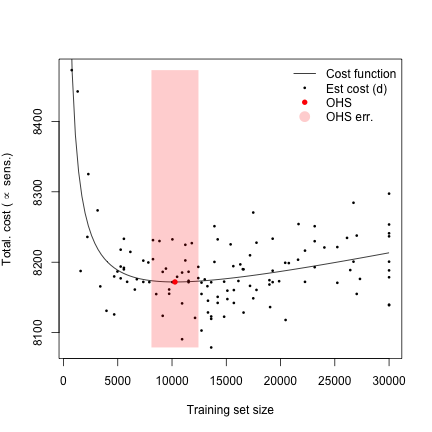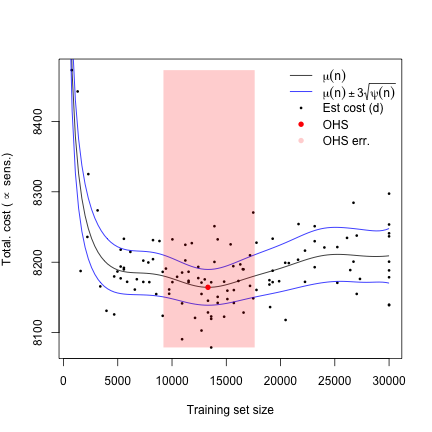## Introduction

In this vignette, we demonstrate the practical estimation of an optimal holdout size for a real predictive model. Since actual training data for this model is unavailable, we estimate an optimal holdout size by simulating the underlying dataset according to published parameters.

Pre-eclampsia (PRE) is a serious hypertensive complication of pregnancy typically occurring in the third trimester. It confers a serious risk to the mother and fetus. Treatment with daily aspirin in the second and third trimesters reduces PRE risk [@rolnik17] but universal treatment is contraindicated (@lefevre14, @acog16) suggesting that prediction of PRE has potential use in directing treatment. The ASPRE score (@wright12, @akolekar13) was developed to predict PRE and is useful in this regard [@rolnik17b]. Should the ASPRE score be updated, care will need to be taken to avoid loss of accuracy from score-driven intervention (@lenert19, @sperrin19, @liley20). One simple way to do this is with a hold-out set.

An ethical difficulty arises in that individuals in this hold-out set would lose any benefit of the ASPRE score, and if the hold-out set is small, the score will be inaccurate and of limited future use.

## Estimation of optimal holdout size

### Parameters $$N$$ and $$k_1$$

We suppose that ASPRE is to be refitted five-yearly. The total set of samples on which a new score must be developed, and then can be used on, comprises all pregnancies over five years. We presume that the score is to be used and fitted in a country or region of population 5 million, from which there will be approximately 80,000 new pregnancies per year. Now $$N\approx 400,000$$, with standard error around 1500 (derived from the standard deviation of a binomial proportion).

We that intervention comprises treating the highest-risk $$\pi=10%$$ of individuals with aspirin. Amongst untreated patients, we denote $$\pi_0$$ as the proportion of 'low-risk' individuals (not in highest 10% risk) who will develop PRE, and $$\pi_1$$ as the proportion of 'high-risk' (highest 10% risk) who will develop PRE (noting that $$\pi_0$$ and $$\pi_1$$ depend on the accuracy of the risk score determining risk quantiles).

Using the current best practice according to ACOG guidelines [@ogorman17], we have $$\pi_0 \approx 0.02$$ (SE $$0.0009$$) and $$\pi_1 \approx 0.08$$ (SE $$0.008$$). The reduction in PRE risk with aspirin treatment is approximately $$1-\alpha=63\%$$ (SE 0.09) [@rolnik17], which we assume is independent of PRE risk. We take 'cost' to simply be the number of PRE cases in a population, or equivalently average PRE risk. The expected cost per individual under 'baseline' treatment ($$k_1$$) is thus

$k_1 = \pi_0(1-\pi) + \pi_1 \pi \alpha \approx 0.022$

with standard error approximately 0.001 (see manuscript and supplement for details)


# Set random seed
seed=463825
set.seed(seed)

# Libraries
library(mvtnorm)
library(matrixStats)
library(mle.tools)
library(OptHoldoutSize)

# Save plot to file, or not
save_plot=FALSE

# Force redo: set to TRUE to regenerate all datasets from scratch
force_redo=FALSE

#### ASPRE-related settings

# Total individuals in trial; all data
n_aspre_total=58794

# Population untreated PRE prevalence
pi_PRE = 1426/58974

# Maximum score sensitivity amongst highest 10%: assumed to be that of ASPRE
sens_max = (138+194)/2707  # = 0.122645 , from abstract of Rolnik 2017 Ultrasound in O&G

# Intervene with aspirin on this proportion of individuals
pi_intervention=0.1

# Aspirin reduces PRE risk by approximately this much
alpha=0.37
SE_alpha=0.09

# Candidate values for n
nval=round(seq(500,30000,length=100))

# Parameter calculation for N
N=400000; SE_N=1500

# Parameter calculation for k1
NICE_sensitivity=0.2
pi_1=NICE_sensitivity*(239/8875)/pi_intervention
pi_0=(1-NICE_sensitivity)*(239/8875)/(1-pi_intervention)
SE_pi_1=sqrt(pi_1*(1-pi_1)/(8875*0.1))
SE_pi_0=sqrt(pi_0*(1-pi_0)/(8875*0.9))
k1=pi_0*(1-pi_intervention) + pi_1*pi_intervention*alpha

# Standard error for k1
pi_1_s=rnorm(1000,mean=pi_1,sd=SE_pi_1)
pi_0_s=rnorm(1000,mean=pi_0,sd=SE_pi_0)
alpha_s=rnorm(1000,mean=alpha,sd=SE_alpha)
SE_k1=sd(pi_0_s*(1-pi_intervention) + pi_1_s*pi_intervention*alpha_s)



### Simulation of dataset

We simulate a dataset of covariates $$X$$ and outcome $$Y$$ (where $$Y$$ indicatesPRE status under baseline treatment) with distribution of $$X$$ as specified in @rolnik17. We take the ASPRE model as ground-truth for $$P(Y=1|X)$$ up to a linear transformation of the argument of the link function, which we choose such that the population prevalence of PRE and the expected sensitivity of the score amongst the highest-risk 10% of individuals matches that reported in @rolnik17.

# Parameters of true ASPRE dataset
data(params_aspre)

# Simulate random dataset
X=sim_random_aspre(n_aspre_total,params=params_aspre)

# Risk will be monotonic to ASPRE risk, but we will transform to match
#  population prevalence of PE and sensitivity of ASPRE score.
risk0=aspre(X1)

# Find a linear transformation ax+b of lrisk such that population prevalence
#  and expected sensitivity match. Suppose P(Y_i=1)=score_i
# Expected sensitivity = E_{Y|scores}(sens)
#                      = (1/(pi_intervention*n_aspre_total))*E{sum_{i:score(i)>thresh} [Y_i]}
#                      = (1/5879)*sum_{i:score(i)>thresh} [(score(i)])
lrisk0=logistic(risk0)
f_ab=function(ab) {
a=ab; b=ab
risk_ab=a*lrisk0 + b
pop_prev=mean(logit(risk_ab))
q_pi=quantile(risk_ab,0.9)
sens=(1/(pi_intervention*n_aspre_total))*sum(logit(risk_ab)*(risk_ab>q_pi))
return((pop_prev-pi_PRE)^2 + (sens - sens_max)^2)
}
abmin=optim(c(1,0),f_ab)$par lrisk=abmin*lrisk0 +abmin risk=logit(lrisk) # PRE is a 0/1 variable indicating whether that simulated patient had PRE. PRE=rbinom(n_aspre_total,1,prob=risk) # ASPRE=ground truth  ### Computation of OHS using parametric method We compute cost as the expected number of cases given a risk score of a particular accuracy. With our assumptions, this can be done readily. However, for demonstration's sake, we select 120 values of $$n$$ at which to calculate $$k_2(n)$$, the expected cost to an individual given a risk score fitted to $$n$$ samples. (not run) Show code set.seed(487276) # Start with estimates of k2 at 10 values of n nn_par=round(runif(20,20,150)^2) k2_par=0*nn_par; for (i in 1:length(nn_par)) { k2_par[i]=aspre_k2(nn_par[i],X,PRE) } # Candidate values for n nval=round(seq(500,30000,length=100)) # Starting value for theta theta=powersolve_general(nn_par,k2_par)$par
theta_se=powersolve_se(nn_par,k2_par,init=theta)

# Rough estimate for variance of k2
dvar0=var(k2_par-powerlaw(nn_par,theta))
s2_par=rep(dvar0,length(k2_par))

for (i in 1:100) {
nxn=next_n(nval,nn_par,k2_par,var_k2 = s2_par,N=N,k1=k1,nmed=10)
if (any(is.finite(nxn))) n_new=nval[which.min(nxn)] else n_new=sample(nval,1)
k2_new=aspre_k2(n_new,X,PRE)
nn_par=c(nn_par,n_new)
k2_par=c(k2_par,k2_new)
s2_par=c(s2_par,dvar0)
print(i)
}

# Resample k2(n), to avoid double-dipping effect
for (i in 1:length(nn_par)) {
k2_par[i]=aspre_k2(nn_par[i],X,PRE)
}

# Transform to total cost
cc_par=k1*nn_par + k2_par*(N-nn_par)

# Save
aspre_parametric=list(nn_par=nn_par,k2_par=k2_par,s2_par=s2_par,cc_par=cc_par)
save(aspre_parametric,file="data/aspre_parametric.RData")



We begin by estimating power-law parameters $$\theta=(a,b,c)$$ with $$k_2(n;\theta)=a n^{-b} + c$$, and associated standard error covariance matrix:

# Load data
data(aspre_parametric)
for (i in 1:length(aspre_parametric)) assign(names(aspre_parametric)[i],aspre_parametric[[i]])

theta=powersolve_general(nn_par,k2_par)$par theta_se=powersolve_se(nn_par,k2_par,init=theta) print(theta) #>  0.34716680 0.88863116 0.02025347 print(theta_se) #> [,1] [,2] [,3] #> [1,] 2.080640e-01 6.843746e-02 1.206067e-05 #> [2,] 6.843746e-02 2.266348e-02 4.095886e-06 #> [3,] 1.206067e-05 4.095886e-06 9.012009e-10  We can now estimate the optimal holdout size, minimum total cost, and confidence interval  # Optimal holdout set size and cost optim_aspre=optimal_holdout_size(N,k1,theta) OHS_ASPRE=optim_aspre$size
MIN_COST_ASPRE=optim_aspre$cost # Errors cov_par=matrix(0,5,5); cov_par[1,1]=SE_N^2; cov_par[2,2]=SE_k1^2 cov_par[3:5,3:5]=theta_se CI_OHS_ASPRE=ci_ohs(N,k1,theta,sigma=cov_par,mode = "asymptotic",grad_nstar=grad_nstar_powerlaw,alpha = 0.1) print(round(OHS_ASPRE)) #>  10271 print(round(MIN_COST_ASPRE)) #>  8172 print(round(CI_OHS_ASPRE)) #> lower upper #> 8103 12438  The following plot shows the estimated cost function, along with estimates of $$n$$ and $$L(n)=k_1 n + k_2(n;\theta)(N-n)$$.Show code  plot(0,xlim=range(nn_par),ylim=range(cc_par),type="n", xlab="Training set size", ylab=expression(paste("Total. cost ", "(", "","", phantom() %prop% phantom(), " sens.", ")", ""))) points(nn_par,cc_par,pch=16,cex=0.5) lines(nval,k1*nval + powerlaw(nval,theta)*(N-nval)) e_min=min(CI_OHS_ASPRE); e_max=max(CI_OHS_ASPRE); c_min=min(cc_par); c_max=max(cc_par); polygon(c(e_min,e_min,e_max,e_max),c(c_min,c_max,c_max,c_min), col=rgb(1,0,0,alpha=0.2),border=NA) points(OHS_ASPRE,MIN_COST_ASPRE,pch=16,col="red") legend("topright", c("Cost function", "Est cost (d)", "OHS", "OHS err."), lty=c(1,NA,NA,NA),lwd=c(1,NA,NA,NA),pch=c(NA,16,16,16),pt.cex=c(NA,0.5,1,2), col=c("black","black","red",rgb(1,0,0,alpha=0.2)),bg="white",border=NA) if (save_plot) dev.off()  ### Computation of OHS using emulation method Alternatively, we may use the emulation method. This would be particularly advised if a complex machine learning algorithm was being used to fit the predictive score, in which case the function $$k_2$$ may not be readily parametrisable. We begin by setting variance and covariance parameters # Variance and covariance parameters var_u=1000 k_width=5000  We proceed as for the parametric method by successively selecting new values of $$n$$ at which to estimate $$k_2(n)$$. (not run) Show code  # Begin as for parametric approach set.seed(487276) # Start with estimates of k2 at 10 values of n nn_emul=round(runif(20,20,150)^2) k2_emul=0*nn_emul; for (i in 1:length(nn_emul)) { k2_emul[i]=aspre_k2(nn_emul[i],X,PRE) } # Candidate values for n nval=round(seq(500,30000,length=100)) # Starting value for theta theta=powersolve_general(nn_emul,k2_emul)$par

# Rough estimate for variance of k2
dvar0=var(k2_emul-powerlaw(nn_emul,theta))
s2_emul=rep(dvar0,length(k2_emul))

for (i in 1:100) {
nxn = exp_imp_fn(nval,nset=nn_emul,k2=k2_emul,var_k2=s2_emul,
N=N,k1=k1,theta=theta,var_u=var_u,k_width=k_width)
n_new = nval[which.max(nxn)]
k2_new=aspre_k2(n_new,X,PRE)
nn_emul=c(nn_emul,n_new)
k2_emul=c(k2_emul,k2_new)
s2_emul=c(s2_emul,dvar0)
theta=powersolve_general(nn_emul,k2_emul)\$par
print(c(i,n_new))
}

# Transform estimated k2 to costs
cc_emul=k1*nn_emul + k2_emul*(N-nn_emul)

# Save
aspre_emulation=list(nn_emul=nn_emul,k2_emul=k2_emul,s2_emul=s2_emul,cc_emul=cc_emul)
save(aspre_emulation,file="data/aspre_emulation.RData")



We evaluate the posterior mean and variance for the Bayesian emulator of the cost function, and estimate the optimal holdout size, minimum cost, and a measure of errror:

# Load data
data(aspre_emulation)
for (i in 1:length(aspre_emulation)) assign(names(aspre_emulation)[i],aspre_emulation[[i]])

# Mean and variance of emulator for cost function, parametric assumptions satisfied
p_mu=mu_fn(nval,nset=nn_emul,k2=k2_emul,var_k2 = s2_emul,
theta=theta,N=N,k1=k1,var_u=var_u,k_width=k_width)
p_var=psi_fn(nval,nset=nn_emul,var_k2=s2_emul,N=N,var_u=var_u,k_width=k_width)

OHS_ASPRE=nval[which.min(p_mu)]
MIN_COST_ASPRE=min(p_mu)
OHS_ERR=error_ohs_emulation(nn_emul,k2_emul,var_k2=s2_emul,N=N,k1=k1,alpha=0.1,
var_u=var_u,k_width=k_width,theta=theta)

print(round(OHS_ASPRE))
#>  13313
print(round(MIN_COST_ASPRE))
#>  8164
print(round(range(OHS_ERR)))
#>   9210 17619


The following figure shows the estimated cost function mean and posterior variance, along with an error measure. The error set is defined as the set of all values $$n$$ for which the probability of the true cost at $$n$$ being less than the observed minimum exceeds 0.1, according to the computed posterior distribution of the cost at $$n$$.Show code

plot(0,xlim=range(nn_emul),ylim=range(cc_emul),type="n",
xlab="Training set size",
ylab=expression(paste("Total. cost ", "(", "","",
phantom() %prop% phantom(), " sens.", ")", "")))
points(nn_emul,cc_emul,pch=16,cex=0.5)
lines(nval,p_mu)
lines(nval,p_mu+3*sqrt(pmax(0,p_var)),col="blue")
lines(nval,p_mu-3*sqrt(pmax(0,p_var)),col="blue")
e_min=min(OHS_ERR); e_max=max(OHS_ERR); c_min=min(cc_emul); c_max=max(cc_emul);
polygon(c(e_min,e_min,e_max,e_max),c(c_min,c_max,c_max,c_min),
col=rgb(1,0,0,alpha=0.2),border=NA)
points(OHS_ASPRE,MIN_COST_ASPRE,pch=16,col="red")

legend("topright",
c(expression(mu(n)),
expression(mu(n) %+-% 3*sqrt(psi(n))),
"Est cost (d)",
"OHS",
"OHS err."),
lty=c(1,1,NA,NA,NA),lwd=c(1,1,NA,NA,NA),pch=c(NA,NA,16,16,16),pt.cex=c(NA,NA,0.5,1,1),
col=c("black","blue","black","red",rgb(1,0,0,alpha=0.2)),bg="white",border=NA)

if (save_plot) dev.off()



### Interpretation

Both methods suggest an optimal holdout size of around 10,000, substantially smaller than the original training set size for the ASPRE model. This suggests, as expected, that further training of a model eventually has diminishing returns.

Note that the points selected to optimise estimation using the parametric method tend to be well spread-out, in order to attain a good estimate of parameters. By contrast, the points selected to optimise estimation using the emulation method tend to be close to the minimum, to favour accurate local approximation of the cost function.